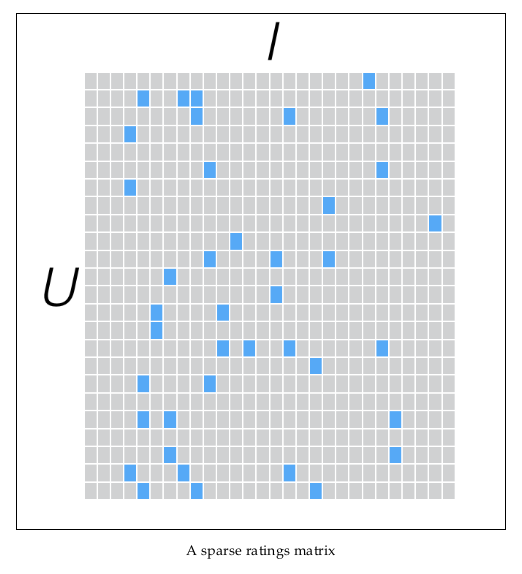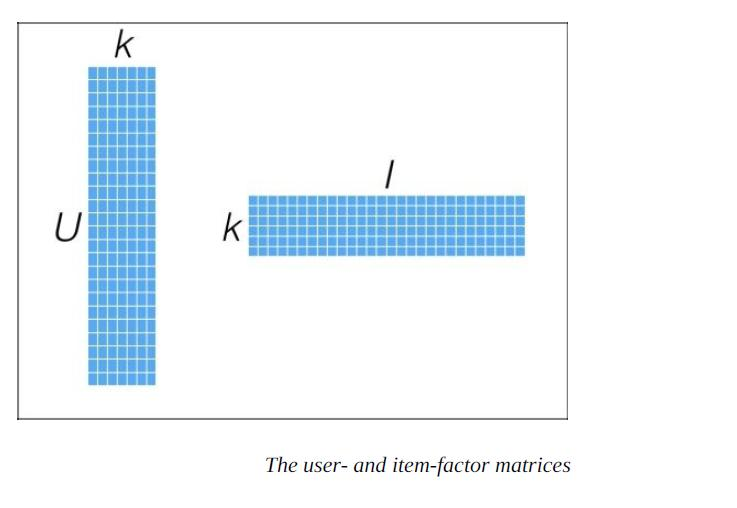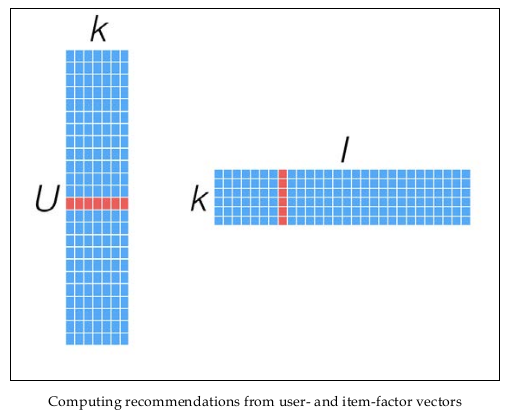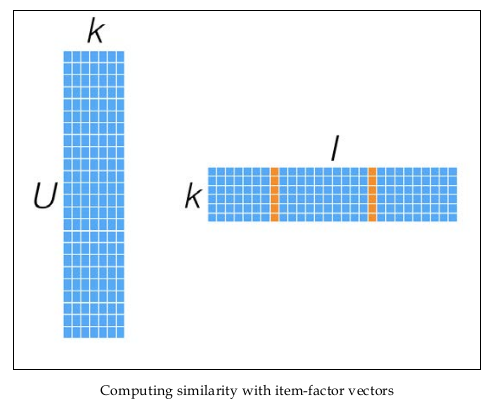# 推荐系统 ALS 算法

ALS（Alternating Least Squares，交替最小二乘法）是矩阵分解方法中对损失函数最小化的一个求解方法，除此之外当然还有其他方法，比如 SVD 等。从协同过滤的分类来说，ALS 算法属于 User-Item CF，也叫做混合 CF，它同时考虑了 User 和 Item 两个方面。

## 矩阵分解

ALS 的矩阵分解算法常应用于推荐系统中，将用户（user）对物品（item）的评分矩阵，分解为用户对物品隐含特征的偏好矩阵物品在隐含特征上的映射矩阵。与传统的矩阵分解 SGD 方法来分解矩阵不同的是，ALS 希望找到两个低维矩阵。用户和物品的关系，可以抽象为如下的三元组：<User,Item,Rating>。其中，Rating 是用户对物品的评分，表明用户对该物品的喜好程度。### 矩阵分解形式

$$R_{m×n} \approx P_{m×k} × Q_{k×n} = \hat{R}_{m×n}$$### 预测模型

$$\sum_{k=1}^{K}p_{i,k}q_{k,j}$$### 损失函数

$$e_{i,j}^2=(r_{i,j}^2-\hat{r}_{i,j}^2)=(r_{i,j}-\sum_{k=1}^{K}p_{i,k}q_{k,j})^2$$

$$min\ L(p_*,q_*)=\sum_{i,j\ is\ known}e_{i,j}^2$$

### 目标函数

$$E_{i,j}^2=(r_{i,j}−∑_{k=1}^{K}p_{i,k}q_{k,j})^2+\frac{β}{2}∑_{k=1}^{K}(p_{i,k}^2+q_{k,j}^2)$$

$$=(r_{i,j}−∑_{k=1}^{K}p_{i,k}q_{k,j})^2+λ∑_{k=1}^{K}|p_{i,k}|^2+λ∑_{k=1}^{K}|q_{k,j}|^2$$

## ALS 算法的优缺点

### ALS 算法的优点

• 在实际应用中，由于待分解的矩阵常常是非常稀疏的，与 SVD 相比，ALS 能有效的解决过拟合问题。
• 基于 ALS 的矩阵分解的协同过滤算法的可扩展性也优于 SVD。
• 与随机梯度下降（SGD）的求解方式相比，一般情况下随机梯度下降比 ALS 速度快；但有两种情况 ALS 更优于随机梯度下降：
• 当系统能够并行化时，ALS 的扩展性优于随机梯度下降法。
• ALS-WR 能够有效的处理用户对商品的隐式反馈的数据。

### ALS 算法的缺点

• 它是一个离线算法，不能体现实时性。
• 无法准确评估新加入的用户或商品，即存在冷启动（cold start）问题。